Published: 30 June 2017

# Adaptive machinery fault diagnosis based on improved shift-invariant sparse coding

Limin Li1
1Xi’an Polytechnic University, Xi’an, China
Views 45

#### Abstract

In machinery fault diagnosis, it is common that one kind of fault may correspond to several conditions, these conditions may contain different loads, different speeds and so on. When using conventional intelligent machinery fault diagnosis methods on diagnosing this kind of faults, if only one condition among all of these conditions was trained, when using this trained classifier for diagnosing fault which containing all conditions, it would obtain a classification result with higher error, it is the problem of robustness; but if we train all these data in each condition, the robustness can be improved a lot, but the time would be wasted. In order to balance these two aspects of fault diagnosis which seem contradict with each other, someone proposed a new method which based on shift-invariant sparse coding (SISC) method, this method can learn features from each condition of the same fault, and these features are adaptive to other conditions, which solve the first problem, but time efficiency of this algorithm is lower, in this paper, by improving the efficiency of shift-invariant sparse coding, we can reduce a lot of time on learning features. Through the experiment testing, it showed that this new method proposed in this paper produced better performance than SISC algorithm.

## 1. Introduction

At present, methods of machinery fault diagnosis can be divided into three categories, they are respectively modeling based methods, signal processing based methods and intelligent diagnosis methods [1-5]. Generally speaking, there are two main steps in fault diagnosis: the first step is feature extraction of vibration signals with some signal processing tools, and the second step is the fault classification based on the extracted features in the previous step [6, 7]. How to extract features from vibration signals is one of the key problem in machine fault diagnosis. Since vibration signals usually contain non-stationary and nonlinear behaviors due to the complexity of the structure and work conditions, it is difficult to achieve an effective fault diagnosis only with the time domain or the frequency domain analysis. Hence features are extracted to indicate the characteristics of vibration signals. The conventional feature extraction methods include time-domain methods, frequency-domain methods, and time-frequency domain methods [8-10]. Features mentioned above are related to the fault, but not all of these features are useful for the classification result, so we have to select some features that are beneficial to the diagnosis result. These two works will not only waste a lot of time, while it would need several evaluation criterions to evaluate the classification result, so it is necessary to change this kind of mode for fault diagnosis.

Especially in the process of fault diagnosis, one problem that need pay attention is that for one machine, one kind of fault may correspond to several conditions, such as different loads, different speeds and so on, for example, inner race fault of a bearing can correspond to 4 different speeds, which would lead to two direct impacts, the first one is that if only one condition among all of these conditions was trained, it would get a classification result with high error while diagnosing other conditions of the same fault, it is the problem of robustness; but if we train all these data in each condition, the robustness can be improved a lot, but it sacrificed the time, because the quantity of the conditions is large.

Recently, much attention has been paid on deep learning algorithm, because of its excellent feature learning ability, especially in the field of image processing  and speech recognition . This kind of algorithm can overcome the disadvantage of traditional feature extraction methods by extracting the sparse representation of raw signal, and this sparse representation are learned from an over-complete dictionary, so it can represent the raw signal ideally. There are also someone who have applied some methods of deep learning on fault diagnosis, Alfredo Kalaitzis etc. proposed a novel convex scheme for simultaneous online fault classification and anomaly detection in a multivariate time-series setting, a sparse representation based approach for recognition of power system transients was proposed by Soumi Chakraborty etc. , Xuefeng Chen etc. proposed a compressed sensing based on dictionary learning for extracting impulse components, which learns a sparse dictionary from a whole noisy signal itself and then employs greedy algorithms to search impulse information in the learned sparse dictionary , a fusion sparse coding algorithm for impulse feature extraction in machinery weak fault detection was proposed by Sen deng etc., firstly, several sparse coding algorithms are executed in parallel independently as participating algorithms, then, fusion scheme of different sparse coding algorithms are presented to improve the accuracy of sparse signal reconstruction, lastly, the proposed method is used to process aircraft engine rotor vibration signals compared with other feature extraction approaches , Nishchal K. Verma etc. proposed a intelligent condition based monitoring method of rotating machines using sparse auto-encoders, sparse auto encoders have the ability to learn good features from the input data in an unsupervised fashion. Sparse auto-encoders and other deep architectures are already showing very good results in text classification, speaker and speech recognition and face recognition as well , Jipeng Xie etc. used deep belief network for learning features from high speed train vibration signals , Lingli Cui etc. proposed a matching pursuit of an adaptive impulse dictionary for bearing fault diagnosis, an adaptive matching pursuit algorithm that uses an impulse dictionary is introduced in this paper for rolling bearing vibration signal processing and fault diagnosis, Haifeng Tang etc. proposed a latent components analysis method based on sparse representation for machinery weak fault detection . Algorithms above and some other paper [21, 22] which using learned sparse dictionary for machinery fault diagnosing, can diagnose different kind of fault with lower correct rate compared to EEMD algorithms, at the same time, it would take a lot of time when data from the same kind of fault which belong to different condition.

In order to solve problems mentioned above, an improve SISC framework for machinery fault diagnosis was proposed, this method can learn useful bases from just one condition of each kind of fault, but it can be adaptive to other conditions, so it can improve the robustness of fault diagnosis, meanwhile, we improved the shift-invariant sparse coding algorithm, which make it more efficient than before.

The remaining sections of this paper are listed as follows: Section 2 described the related work used in this paper, which introduced the algorithm of SISC; the proposed framework for adaptive fault diagnosis was stated in Section 3, which included improved shift-invariant sparse coding and novel framework for adaptive fault diagnosis; experimental test was given in Section 4; at last, we concluded this paper and made some discussion.

## 2. Related work

### 2.1. Shift-invariant sparse coding

SISC algorithm was firstly proposed by Monten Mørup etc., which is an extension of sparse coding which reconstructs one input data using all of the basis functions in all possible shifts .

In order to model SISC, additional parameters need to be introduced into the standard sparse coding model :

1
$X=\sum _{k}{T}_{l}\left({d}_{k}\right){s}_{k,l}+\epsilon ,$

where, $X$ is the input data; ${d}_{k}$ is the $k$th dictionary; ${s}_{k,l}$ is the coefficient associated with the atom ${d}_{k}$ shifted for $l$ offsets; $\epsilon$ is the observation noise with Gaussian distribution; ${T}_{l}$ is a shift operator which shifts an atom for $l\in \left[-L,L\right]$ offsets relatively.

Given a finite set of signals $X=\left\{{X}_{1},{X}_{2},\dots ,{X}_{N}\right\}$, assuming a uniform prior over the basis, a MAP estimator can be formulated for both bases and coefficients:

2
$\underset{d,s}{\mathrm{m}\mathrm{i}\mathrm{n}}\sum _{i=1}^{N}\left({‖{X}_{i}-\sum _{k=1}^{K}{d}_{k}*{s}_{k,i}‖}_{2}^{2}+\beta {\sum _{k=1}^{K}‖{s}_{k,i}‖}_{1}\right),$
$\text{Subject to}\text{}\text{}C\triangleq \left\{{‖{d}_{k}‖}_{2}^{2}\le c,1\le k\le K\right\},$

where $*$ represents convolution operator; ${s}_{k,i}\in {R}^{N-K+1}$ is the sparse activation of ${d}_{k}$ for signal ${X}_{i}$, $N$ is dimension of ${X}_{i}$, $K$ is number of dictionary, $\beta$ is penalty parameter of the sparsity constraint, $C$ is convex set of matrices which preventing ${d}_{k}$ from being very small. Specifically, ${s}_{k,i}$ can be solved in the convex optimization problem with ${d}_{k}$ being held fixed, and vice versa .

When using sparse coding algorithm on machinery fault diagnosing, it can diagnose some kinds of faults, but compared to traditional EEMD algorithm, it has lower correct rate, someone proposed using SISC algorithm to improve the correct rate of fault diagnosing, and gained some improvement , but meanwhile the computation complexity is added, so it is essential to reduce time which took by SISC algorithm, while not reducing the correct rate.

## 3. Proposed framework for adaptive machinery fault diagnosis

Adaptive machinery fault diagnosis algorithm is shown in Fig. 1. In order to reduce the diagnosis time and don’t reduce robustness, we first need to learn dictionary for each kind of faults, then it is essential to combine all learned dictionaries into one over-complete dictionary, after shift invariant processing, by calculating the coefficients, we obtained all new features of each fault, when we need to diagnose a data set to see which fault it belongs, we need firstly to solve sparse representation, which is the new feature representation, using the trained classifier to diagnose the new data.

Fig. 1Novel framework for machinery fault diagnosis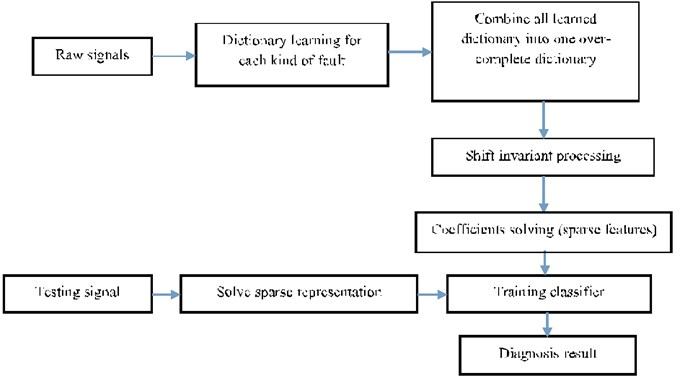In order to improve the computation performance of shift-invariant sparse coding algorithm, using the idea in literature  to solve the problem of SISC algorithm. Firstly, which holding ${d}_{k}$ fixed, solving ${s}_{k,i}$ by Eq. (3). Using feature-sign search algorithm for solving:

3
$\underset{{s}_{k,i}}{\mathrm{m}\mathrm{i}\mathrm{n}}{\sum }_{i=1}^{N}\left({‖{X}_{i}-{\sum }_{k=1}^{K}{d}_{k}*{s}_{k,i}‖}_{2}^{2}+\beta {\sum }_{k}\left|{s}_{k,i}\right|\right).$

Feature-sign search algorithm used for SISC:

(1) Initialize ${s}_{k}:=\stackrel{\to }{0}$, $\theta :=\stackrel{\to }{0}$, and “active set” $:=\left\{\right\}$, where ${\theta }_{i}\in \left\{-1,0,1\right\}$ denotes sign (${s}_{k,i}$).

(2) From zero coefficients of ${s}_{k}$, select $i=\mathrm{a}\mathrm{r}\mathrm{g}ma{x}_{i}\left|\frac{\partial {‖{X}_{i}-{d}_{k}*{s}_{k}‖}^{2}}{\partial {s}_{k,i}}\right|$, active ${s}_{k,i}$ (add $i$ to “active set”) only if locally improves the objective, namely:

• If $\frac{\partial {‖{X}_{i}-{d}_{k}{s}_{k}‖}^{2}}{\partial {s}_{k,i}}>\beta$, then set ${\theta }_{i}:=-1$, “active set” $:=\left\{i\right\}\cup$ “active set”.

• If $\frac{\partial {‖{X}_{i}-{d}_{k}*{s}_{k}‖}^{2}}{\partial {s}_{k,i}}<-\beta$, then set ${\theta }_{i}:=1$, “active set” $:=\left\{i\right\}\cup$ “active set”.

(3) Feature-sign step: Set $A={\sum }_{k=1}^{K}{d}_{k}$, let $\stackrel{^}{A}$ be a sub-matrix of $A$ that contains only the columns corresponding to the “active set”.

Let ${\stackrel{^}{s}}_{k,i}$ and $\stackrel{^}{\theta }$ be sub-vector of ${s}_{k}$ and $\theta$ corresponding to the “active set”.

Compute the analytical solution to the resulting unconstrained QP ($\mathrm{m}\mathrm{i}\mathrm{n}\mathrm{i}\mathrm{m}\mathrm{i}\mathrm{z}\mathrm{e}\mathrm{}{\stackrel{^}{s}}_{k}{‖{X}_{i}-{d}_{k}{s}_{k}‖}^{2}+\beta {\stackrel{^}{\theta }}^{T}{\stackrel{^}{s}}_{k}$):

${\stackrel{^}{s}}_{k,new}:=\left({\stackrel{^}{A}}^{T}\stackrel{^}{A}{\right)}^{-1}\left({\stackrel{^}{A}}^{T}{X}_{i}-\beta \stackrel{^}{\theta }/2\right)$,

Perform a discrete line search on the closed line segment from ${\stackrel{^}{s}}_{k}$ to ${\stackrel{^}{s}}_{k,new}$:

Check the objective value at ${\stackrel{^}{s}}_{k,new}$ and all points where any coefficient changes sign.

Update ${\stackrel{^}{s}}_{k}$(and the corresponding entries in ${s}_{k}$) to the point with the lowest objective value.

Remove zero coefficients of ${\stackrel{^}{s}}_{k}$ from the active set and update $\theta :=sign\left(x\right)$.

(4) Check the optimality conditions: (a) Optimality condition for nonzero coefficients: $\frac{\partial {‖{X}_{i}-{d}_{k}{s}_{k}‖}^{2}}{\partial {s}_{k,j}}+\beta sign\left({s}_{k,j}\right)=0$, $\forall {s}_{k,j}\ne 0$.

If condition (a) is not satisfied, go to step (3); else check condition (b).

(b) Optimality condition for zero coefficients: $\frac{\partial {‖{X}_{i}-{d}_{k}{s}_{k}‖}^{2}}{\partial {s}_{k,j}}\le \beta$, $\forall {s}_{k,j}=0$.

If condition (b) is not satisfied, go to step (2); otherwise return ${s}_{k}$ as the solution.

Then holding ${s}_{k,i}$ fixed, solve ${d}_{k}$ by Eq. (4). Using the Lagrange dual for solving :

4
$\underset{{d}_{k}}{\mathrm{m}\mathrm{i}\mathrm{n}}{‖{X}_{i}-{\sum }_{k=1}^{K}{d}_{k}*{s}_{k,i}‖}_{2}^{2},$
$\text{Subject to}\mathrm{}\mathrm{}\sum _{k=1}^{K}{{d}_{k}}^{2}\le c,1\le k\le K.$

## 4. Experimental testing

### 4.1. Experimental set-up

Data used in this paper for experimental testing are provided by Case Western Reserve University (CWRU), the basic layout of the test rig is shown in Fig. 2. It consists of a 2 hp reliance electric motor driving a shaft on which a torque transducer and encoder are mounted. Torque is applied to the shaft via a dynamo-meter and electronic control system. For these tests, faults ranging in diameter from 0.007 to 0.028 in. (0.18-0.71 mm) were seeded on the drive-end and fan-end bearings (SKF deep-groove ball bearings: 6205-2RSJEM and 6203-2RSJEM, respectively) of the motor using electro-discharge machining (EDM). The faults were seeded on the rolling elements and on the inner and outer races, and each faulty bearing was reinstalled (separately) on the test rig, which was then run at constant speed for motor loads of 0-3 horse power (approximate motor speeds of 1720-1797 rpm). Further details regarding the test set-up can be found at the CWRU Bearing Data Center website .

In order to test the improved SISC algorithm, 16 classes data were used, which include data in normal condition and in three fault condition, the used data are shown in Table 1 and Table 2.

Fig. 2CWRU bearing test rig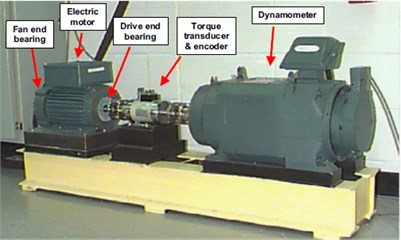Table 1Training data used in this paper (–: None)

 Fault diameter (in) Load Speed Inner race Ball Outer_6 Outer_3 Outer_12 0.007 0 1797 IR007_0 B007_0 OR007_6_0 OR007_3_0 OR007_12_0 0.014 0 1797 IR014_0 B014_0 OR014_6_0 – – 0.021 0 1797 IR021_0 B021_0 OR021_6_0 OR021_3_0 OR021_12_0 0.028 0 1797 IR028_0 B028_0 – – – 0 (N000, Normal) 0 1797 – – – – –

Table 2Testing data used in this paper

 Fault diameter (in) Load Speed Inner race Ball Outer_6 Outer_3 Outer_12 0.007 1 1772 IR007_1 B007_1 OR007_6_1 OR007_3_1 OR007_12_1 2 1750 IR007_2 B007_2 OR007_6_2 OR007_3_2 OR007_12_2 3 1730 IR007_3 B007_3 OR007_6_3 OR007_3_3 OR007_12_3 0.014 1 1772 IR014_1 B014_1 OR014_6_1 OR014_3_1 OR014_12_1 2 1750 IR014_2 B014_2 OR014_6_2 OR014_3_2 OR014_12_2 3 1730 IR014_3 B014_3 OR014_6_3 OR014_3_3 OR014_12_3 0.021 1 1772 IR021_1 B021_1 OR021_6_1 OR021_3_1 OR021_12_1 2 1750 IR021_2 B021_2 OR021_6_2 OR021_3_2 OR021_12_2 3 1730 IR021_3 B021_3 OR021_6_3 OR021_3_3 OR021_12_3 0.028 1 1772 IR028_1 B028_1 OR028_6_1 OR028_3_1 OR028_12_1 2 1750 IR028_2 B028_2 OR028_6_2 OR028_3_2 OR028_12_2 3 1730 IR028_3 B028_3 OR028_6_3 OR028_3_3 OR028_12_3

### 4.2.1. Training results

We use half data of load 0 for calculating dictionary representation and its corresponding coefficient, and use algorithm proposed in this paper for training, and at last corresponding dictionaries of each training data were calculated, which are shown in Fig. 3.

Dictionaries calculated by improved SISC algorithm are shown in Fig. 3, sparse features of 16 kinds of bearing faults were extracted, each dictionary composed of 8 bases, this series of dictionaries indicate different vibration signal can be represented by different dictionaries. Coefficients of each base composed of the sparse features, Table 3 is the classification error rate of training features extracted by SISC algorithm using SVM algorithm.

Fig. 3Raw signal of 2 of 16 kinds of bearing faults and their corresponding dictionary with one dictionary set of 204 shift variable dictionary and W:204×8×204, (length of dictionary * number of dictionaries * shift time) x-axis of dictionary: length of the base; y-axis of dictionary: amplitude of base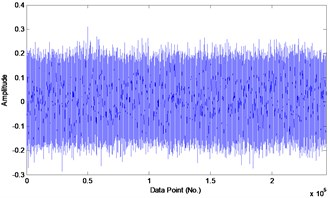a) Data 1: (N000, Normal)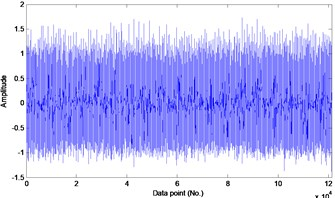b) Data 2: (IR007_0)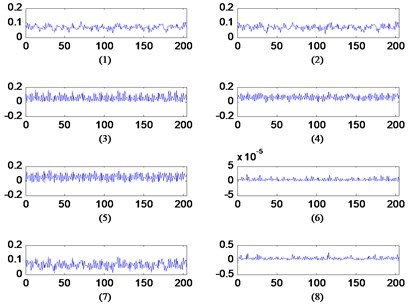c) Data 1: Dictionary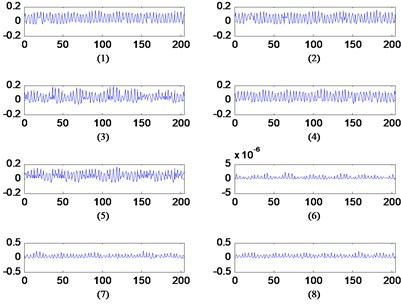d) Data 2: Dictionary

Table 3Classification error rate of training data (–: None)

 Fault diameter (in) Classification error rates (%) Normal Inner race ball Outer race position Center @6:00 Orthogonal @3:00 Opposite @12:00 0 0 – – – – – 0.007 – 0.36 0.57 0 0 0.09 0.014 – 0 1.86 1.2 – – 0.021 – 0.96 0.89 3.75 0 0.49 0.028 – 1.24 1.76 – – –

### 4.2.2. Recognition correct rate between SISC and improved SISC algorithm

After calculating dictionary of each kind of fault, we combine all those dictionaries into one over-complete dictionary, there are a variety of method for fusing child dictionaries into one over-complete dictionary according to the needs of signal sparse representation solution, the simplest way is by connecting directly parallel combination more child dictionaries :

5
$D=\left[{d}_{1},{d}_{2},\dots ,{d}_{k}\right],$

where ${d}_{k}$ is child dictionary, $D$ is over-complete dictionary. Connect a parallel combination more child dictionaries to one to solve the coefficient of new kind of fault, it is easy to diagnosis the fault class by training classifier with sparse represent, which can be called sparse features, using all these sparse features to train classifier, and took other half data as testing data, in this paper, SVM classifier was used . In order to compare recognition correct rate between SISC and improved SISC algorithm, using features extracted by SISC and improved SISC algorithm for testing, Table 4 are classification error rate of 60 groups test data.

From Table 4 we can conclude that when using improved SISC for classing machinery fault, the classification error rate is smaller than results which using SISC algorithm, so we can gain the conclusion that algorithm proposed in this paper improved the adaptive performance of SISC algorithm for fault diagnosing.

Table 4Classification error rate of testing data between SISC and improved SISC (–: None; _1: Improved SISC, _2: SISC)

 Fault diameter (in) Load (Hp) Classification error rates (%) Inner race Ball Outer race position Center @6:00 Orthogonal @3:00 Opposite @12:00 0.007 1_1 0 3.79 0.76 2.98 0 1_2 30.00 50.22 25.78 34.87 55.13 2_1 0 1.78 0 1.67 0 2_2 60.54 44.76 55.34 23.20 34.76 3_1 1.79 3.66 0 2.45 0 3_2 30.45 24.56 44.63 43.72 50.07 1_1 0.56 2.36 4.97 – – 1_2 22.78 60.79 22.54 – – 0.014 2_1 20.65 2.98 0.65 – – 2_2 67.65 33.52 32.16 – – 3_1 40.20 1.88 2.76 – – 3_2 60.98 23.43 44.72 – – 1_1 1.34 23.26 5.47 0.78 5.63 1_2 23.15 45.23 50.12 64.23 54.16 0.021 2_1 0.34 20.05 27.62 0 0 2_2 33.75 55.32 67.83 53.12 12.34 3_1 3.78 20.08 25.09 1.54 1.32 3_2 45.20 67.39 30.65 44.91 27.63 1_1 1.76 4.02 – – – 1_2 43.78 23.89 – – – 0.028 2_1 4.89 4.50 – – – 2_2 46.72 52.19 – – – 3_1 1.87 10.34 – – – 3_2 22.19 70.52 – – –

Table 5The running time (in seconds) for shift-invariant sparse coding algorithm and improved shift-invariant sparse coding algorithm for fault diagnosis (1: SISC, 2: improved SISC)

 Fault diameter (in) Load (Hp) Different algorithms Running time (s) Inner race Ball Outer race position Center @6:00 Orthogonal @3:00 Opposite @12:00 0.007 1 1 1.79 1.64 1.75 2.05 3.65 1 2 0.85 0.83 0.88 1.76 3.07 2 1 1.89 1.93 2.33 2.88 2.23 2 2 0.87 0.72 1.90 1.34 1.02 3 1 1.90 1.88 2.35 2.98 1.89 3 2 0.88 0.75 1.80 1.76 0.34

### 4.2.3. Computation complexity

At the same time, the improved SISC algorithm has improved the efficiency of raw SISC algorithm, the running time (in seconds) for shift-invariant sparse coding algorithm and improved shift-invariant sparse coding algorithm has been listed in Table 5. In Table 5, we listed the running time of testing data 0.007 (fault diameter (in)), which include 15 group testing data.

From Table 5 we can conclude that the running time of improved shift-invariant sparse coding algorithm is less than the algorithm of shift-invariant sparse coding.

## 5. Conclusions

In this paper, in order to improve the adaptive performance and reduce the time used for training SISC, an improved algorithm was proposed, which using feature-sign search algorithm and Lagrange dual for solving the dictionaries and coefficients of SISC model, by experimental testing, we can obtain the result that improved SISC can meet the need of adaptive and computation complexity for fault diagnosis, which change the conventional fault diagnosis model, and obtain a better result. It improves the performance of machinery fault diagnosis in two aspect, the first one is that according to the machinery fault diagnosis in multi-condition, adaptive machinery fault diagnosis algorithm was proposed, and another one is that the computation performance SISC was improved. But there are still some problems we need to deeply consider, such as the diagnosis accuracy in this adaptive condition, although it is not a key problem at present.

#### Cited by

Dual-kernel driven convolutional sparse learning for bearing transient feature enhancement

18 August 2016
Accepted
12 March 2017
Published
30 June 2017
SUBJECTS
Fault diagnosis based on vibration signal analysis
Keywords